# Biot-Savart law

In physics, more particularly in electrodynamics, the law first formulated by Jean-Baptiste Biot and Félix Savart  describes the magnetic induction B (proportional to the magnetic field H) caused by a direct electric current in a wire. Biot and Savart interpreted their measurements by a simple algebraic relation. Laplace gave a differential form of their result, which now is often referred to as the Biot-Savart law, or sometimes as the Biot-Savart-Laplace law. By integrating Laplace's equation over an infinitely long wire, the original equation of Biot and Savart is obtained.

## Ørsted's discovery

The Danish physicist Ørsted noticed in April 1820, while experimenting with the Voltaic pile, an effect of an electric current on a permanent magnet. He wrote a Latin publication which he sent round Europe. François Arago demonstrated the discovery at the French Académie des Sciences (11 September 1820), which inspired Jean-Baptiste Biot and André-Marie Ampère to investigate the effect further. At a meeting of the Académie des Sciences on 30 October 1820, Biot and Savart announced that the magnetic force exerted by an infinitely long conductor on a magnetic pole falls off with the reciprocal of the distanceand is orientated perpendicular to the wire. Simultaneously they published this in a short note. Laplace generalized their result mathematically.

## Laplace's formula

At point r the magnetic induction dB due to ids (see the figure on the right and note that for typographical reasons vector arrows are omitted in running text) is given by the following formula due to Laplace,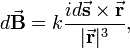$d\vec{\mathbf{B}} = k \frac{i d\vec{\mathbf{s}} \times \vec{\mathbf{r}}} {|\vec{\mathbf{r}}|^3},$

where the magnetic induction is given as a vector product, i.e., is perpendicular to the plane spanned by ds and r. The direct electric current i is constant in time. The piece of the wire contributing to the magnetic induction B can be seen as a vector of infinitesimal length ds and with direction tangent to the wire. The constant k depends on the units chosen. In SI units k is equal to μ0/(4π)—the magnetic constant μ0 divided by 4π—and μ0 = 4π ×10−7 N/A2 (newton over ampere squared). In Gaussian units k = 1/c (one over the speed of light).

The vector r having dimension length, Laplace's formula describes an Inverse-square law.

## Infinite straight conductor

Take a straight, infinitely long, conducting wire transporting the direct current i. Historically, this is the case considered by Biot and Savart. The magnetic field H at a distance R from the wire is (in SI units)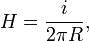$\qquad H = \frac{i}{ 2\pi R},$

where in vacuum the magnetic field and the magnetic induction are related by B = μ0H (SI units) or B = H (Gaussian units).

In order to prove the Biot-Savart law, one writes, using R = r sinα (see the figure),$d\vec{\mathbf{s}} \times \vec{\mathbf{r}} = \hat{\mathbf{e}} \,r\sin\alpha\, ds = \hat{\mathbf{e}}\, R\,ds,$

where$\hat{\mathbf{e}}$ is a unit vector along B, which is perpendicular to the plane spanned by the wire and the vector R perpendicular to the wire. Note that if ds is moved along the wire, all contributions from the segments to the magnetic induction are along this unit vector. Hence, upon integration over the wire all these contributions are added, so that$\vec{\mathbf{B}} = \hat{\mathbf{e}} \,i R k \int_{-\infty}^{\infty} \frac{ds}{(s^2+R^2)^{3/2}}$

where, by the Pythagorean theorem,$|\vec{\mathbf{r}}|^2 = s^2 + R^2.$

Substitution of y = s / R and y = cotφ = cosφ / sinφ, successively, gives finally,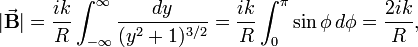$|\vec{\mathbf{B}}| = \frac{ik}{R} \int_{-\infty}^{\infty} \frac{dy}{(y^2+1)^{3/2}} = \frac{ik}{R} \int_{0}^{\pi} \sin\phi \, d\phi = \frac{2 ik}{R},$

where i is the current and R is the distance of the point of observation to the wire (length of vector R). The constant k depends on the choice of electromagnetic units and is equal to μ0/4π (= 10−7 henry/m) in SI units. In Gaussian units k = 1/c. This equation gives the original formulation of Biot and Savart.

The SI dimension of B is T (tesla). A field of 1 T corresponds to 10 000 G (gauss).

## Magnetic field on axis of circular currentA current i runs on circle of radius r in the x-y plane. At a distance R of the center there is a magnetic induction B along the z-axis.
The Biot-Savart-Laplace expression can be integrated in cylinder coordinates for a point R on the z-axis. This gives (see figure on the right) a vector parallel to the z-axis of magnitude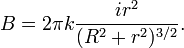$B = 2\pi k \frac{i r^2}{(R^2+r^2)^{3/2}}.$

Note that the direction of the electric current i and the magnetic induction B are related according to the right-hand screw rule: the screw is driven into the direction of B and rotated along i. The factor k is μ0/4π for SI units and 1/c for Gaussian units.

## Generalized Biot-Savart-Laplace law

From hereon vectors are indicated by bold letters, arrows on top are omitted.

In the above we wrote i for the current, which—providing J is constant over the cross section—is equal to the current density J (current per unit area) times the cross section A of the wire. If the current density J is not constant over the cross section, i.e., J = J(r' ), we must use a surface integral over the cross section A$i = \iint_\text{A} \mathbf{J}(\mathbf{r})\cdot d\mathbf{A}.$

Rather than proceeding with the surface element, we multiply by the infinitesimal height ds and obtain an infinitesimal volume element dr′ := dsdA,$i d\mathbf{s} \rightarrow \iint_{A} \mathbf{J}(\mathbf{r}') d\mathbf{r}',$

where we defined the current density J as a vector parallel to the line segment ds and of magnitude J(r'). The volume element has height |ds| = ds and base the infinitesimal surface element dA of the cross section A. The B-field at point r, due to a volume V = As of the current becomes,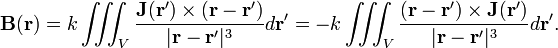$\mathbf{B}(\mathbf{r}) = k \iiint_{V} \frac{\mathbf{J}(\mathbf{r}') \times (\mathbf{r}-\mathbf{r'})} {|\mathbf{r}-\mathbf{r'}|^3}d\mathbf{r'} = -k \iiint_{V} \frac{(\mathbf{r}-\mathbf{r'}) \times \mathbf{J}(\mathbf{r}')} {|\mathbf{r}-\mathbf{r'}|^3}d\mathbf{r'}.$

Note that: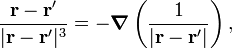$\frac{\mathbf{r}-\mathbf{r'}}{|\mathbf{r}-\mathbf{r'}|^3} = -\boldsymbol{\nabla} \left( \frac{1}{|\mathbf{r}-\mathbf{r'}|} \right),$

where we choose the nabla operator, the gradient, to act on the unprimed coordinates and hence it may be moved outside the integral, giving the following generalized form of the Biot-Savart-Laplace law for the magnetic induction at point r:$\mathbf{B}(\mathbf{r}) = k \boldsymbol{\nabla} \times \iiint_{V} \frac{\mathbf{J}(\mathbf{r}')} {|\mathbf{r}-\mathbf{r'}|}d\mathbf{r}', \qquad\qquad\qquad\qquad\qquad\qquad\qquad(1).$

Here$\boldsymbol{\nabla} \times$ is the curl and V is a finite volume of the current generating the B-field. The total B-field is obtained by having V encompass all current (integrating over all current).

## Consistency with Maxwell equations

The expression for B given in Eq. (1) is a solution of the Maxwell equations with zero displacement current. This is of interest as it confirms the generally accepted notion that the Maxwell equations form a set of postulates for classical electrodynamics. All electrodynamic results, including the Biot-Savart-Laplace law, must be derivable from them. We will show that the latter law can indeed be seen as a consequence of the Maxwell postulates, although Biot, Savart, and Laplace made their discovery some forty years earlier than Maxwell.

The Helmholtz decomposition of B(r) is,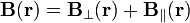$\mathbf{B}(\mathbf{r}) = \mathbf{B}_\perp(\mathbf{r}) + \mathbf{B}_\parallel(\mathbf{r})$

where\begin{align} \mathbf{B}_\perp(\mathbf{r}) &= \frac{1}{4\pi}\boldsymbol{\nabla} \times \iiint \frac{\boldsymbol{\nabla}'\times \mathbf{B}(\mathbf{r}')}{|\mathbf{r}-\mathbf{r}'|} d\mathbf{r}' \\ \mathbf{B}_\parallel(\mathbf{r}) &= -\frac{1}{4\pi}\boldsymbol{\nabla} \iiint \frac{\boldsymbol{\nabla}'\cdot \mathbf{B}(\mathbf{r}')}{|\mathbf{r}-\mathbf{r}'|} d\mathbf{r}' \\ \end{align}

are, respectively, the perpendicular (transverse, divergence-free) and parallel (longitudinal, curl-free) components. The operator acts on unprimed coordinates and ∇' acts on primed coordinates. The pertinent Maxwell's equations are in SI units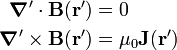\begin{align} \boldsymbol{\nabla}'\cdot \mathbf{B}(\mathbf{r}') &= 0 \\ \boldsymbol{\nabla}'\times \mathbf{B}(\mathbf{r}') & = \mu_0 \mathbf{J}(\mathbf{r}') \end{align}

Hence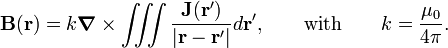$\mathbf{B}(\mathbf{r}) =k \boldsymbol{\nabla} \times \iiint \frac{\mathbf{J}(\mathbf{r}')}{|\mathbf{r}-\mathbf{r}'|} d\mathbf{r}', \qquad\hbox{with}\qquad k= \frac{\mu_0 }{4\pi}.$